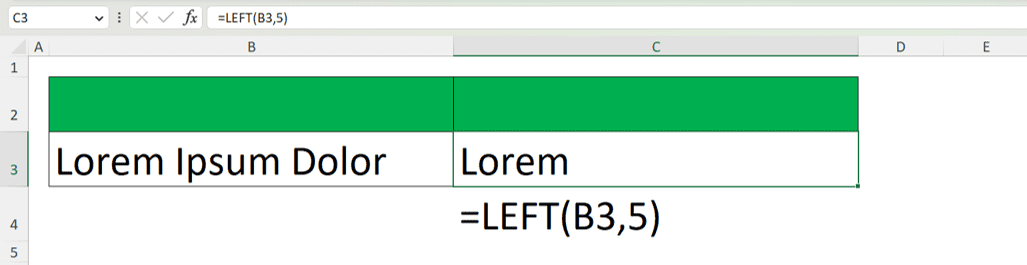Which Function Returns the Leftmost Five Characters in Excel? - Compute Expert

# Which Function Returns the Leftmost Five Characters in Excel?

Home >> Excel Tutorials from Compute Expert >> Excel Tips and Trick >> Excel Quiz >> Which Function Returns the Leftmost Five Characters in Excel?

## Question

Which function returns the leftmost five characters in Excel?
1. =TRIM(B3,5)
2. =RIGHT(B3,5)
3. =MID(B3,5)
4. =LEFT(B3,5)
5. =FIRST(B3,5)

D. =LEFT(B3,5)

## Explanation

Take a look at the screenshot below.As you can see there, the LEFT function can return the leftmost five characters of data in Excel. As the functions in other answer choices cannot do the same, the correct answer here should be D. Here are some examples of what we can call a range in excel.

Go back to the Compute Expert's excel quiz page

Get updated excel info from Compute Expert by registering your email. It's free!